Author: Oscar Cronquist Article last updated on May 05, 2022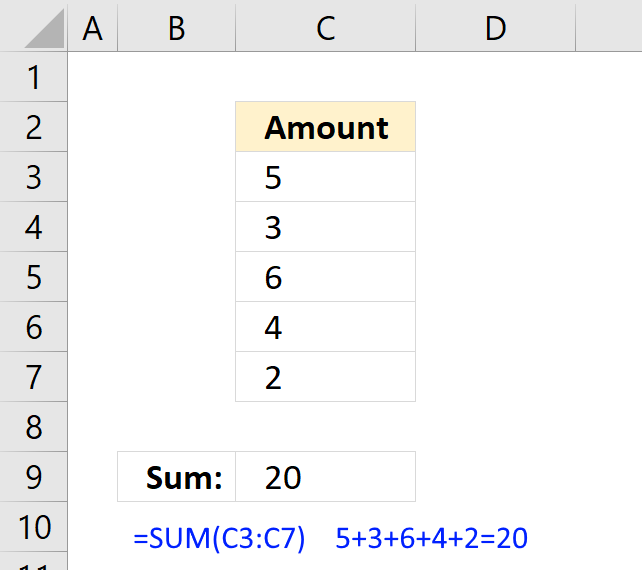The SUM function in Excel allows you to add values, the function returns the sum in the cell it is entered in. The SUM function is cleverly designed to ignore text and boolean values, adding only numbers.

### Excel Function Syntax

SUM(number1, [number2], ...)

#### Arguments

 number1 Required. A constant, cell reference or an array that contains numerical values you want to add. [number2] Optional. Up to 254 additional arguments.

The SUM function lets you add values in cell ranges, arrays, constants. You can have up to 255 different arguments.

## 1. How to add numbers in a column (SUM function)?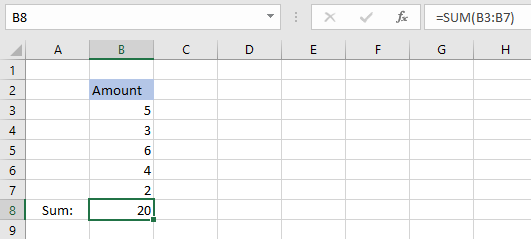The SUM function lets you add values in a cell range, like this = SUM(B3:B7), instead of adding values in a formula using the plus sign, like this =B3+B4+B5+B6+B7.

The SUM function lets you type one or multiple cell ranges, in this example only cell range B3:B7 is entered as an argument. See the above picture.

Check out the shortcut key to automatically sum a column.

## 2. How to add numbers in an array  (SUM function)?

An array is multiple values enclosed with a beginning and ending curly bracket, you can easily convert a cell range to an array. See instructions below.

Select a cell and type =SUM(B3:B9)Press with left mouse button on in the formula bar and select B3:B9.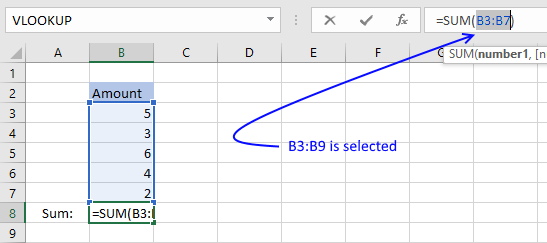Press F9 and the cell range is converted to an array, like this: =SUM({5,3,6,4,2})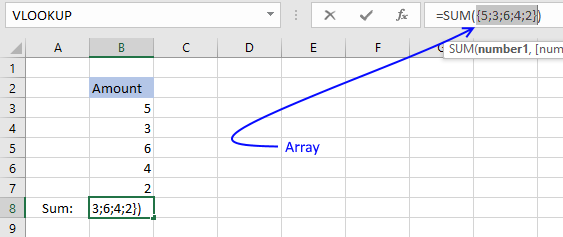Press Enter.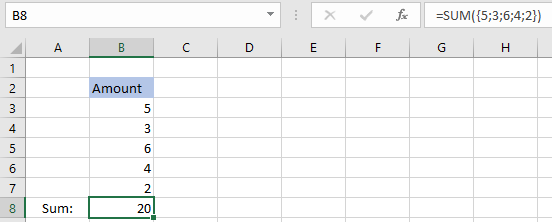The SUM function adds the values in the array 5+3+6+4+2 = 20. When you convert a cell range to values you hard-code or create constants in your formula, meaning they never change unless you change the values in the formula.

Cell references on the other hand change if you change the values on a worksheet.

## 3. How to sum specific cells?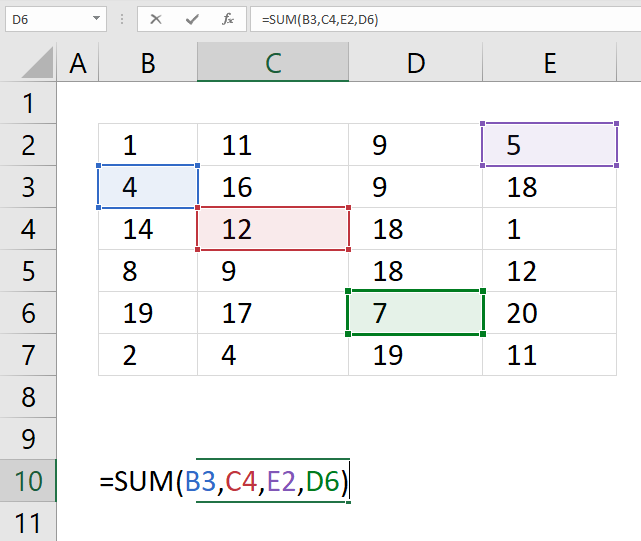The SUM function allows you to add values from the cells you select. The trick is to press and hold the CTRL key while selecting specific cells to sum. Here are the steps in greater detail:

1. Doublepress with left mouse button on a cell, the prompt shows up.
2. Type =SUM(
3. Press and hold CTRL-key.
4. Press with left mouse button on with the left mouse button on cells you want to sum.
5. Release CTRL-key.
6. Add an ending parenthesis )
7. Press Enter.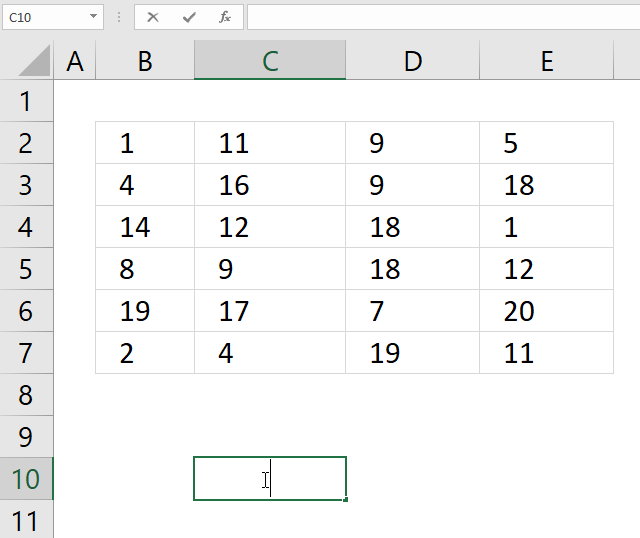## 4. How to sum numbers in multiple cell ranges?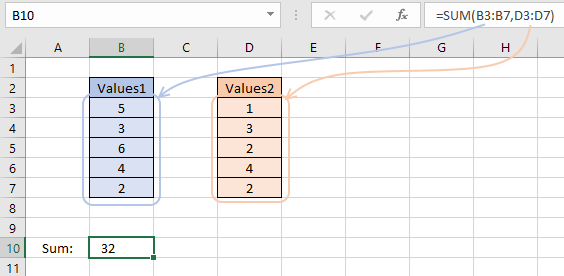If you want to add values in multiple cell ranges you simply use a comma between arguments. Check your regional settings if a comma doesn't work for you. You are allowed to have up to 255 arguments in one SUM function.

1. Doublepress with left mouse button on a cell, the prompt shows up.
2. Type =SUM(
3. Press and hold CTRL-key.
4. Press and hold with the left mouse button.
5. Drag with mouse to select the cell range.
6. Release left mouse button.
7. Go back to step 4 until all cell ranges have been selected.
8. Release CTRL-key.
9. Add an ending parenthesis )
10. Press Enter.

## 5. How to sum a column with text?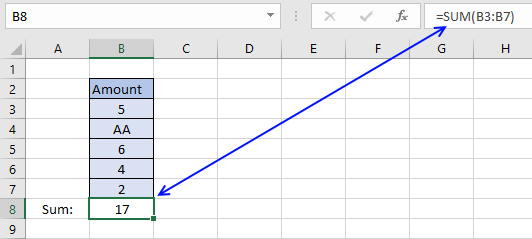The formula in cell B8 adds the values in cell range B3:B7. 5 + AA + 6 + 4 +2 = 17. The SUM function ignores text strings, in this case AA.

The SUM function ignores text values and boolean values but not error values.

Note, the SUM function ignores numbers stored as text. The image below shows the SUM function in cell B8. Only number 4 is included in the total of cells in cell range B3:B7.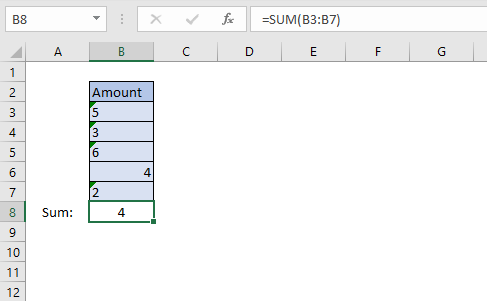Excel shows numbers stored as text differently, see image above. Text values are aligned left in the cell and numbers are aligned right. Cells containing numbers stored as text show a green arrow in the upper left corner of the cell.

## 6. How to sum boolean values?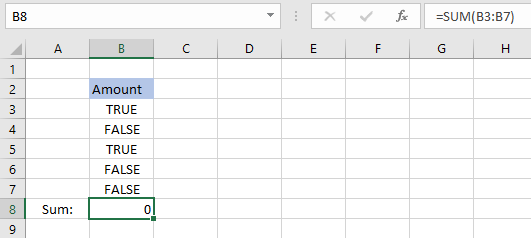Cell range B3:B7 contains boolean values, TRUE or FALSE, however, the SUM function can't add boolean values unless they are converted to their numerical equivalents.

There are multiple solutions to this problem, here are a few:

Formula in cell B8:

=SUM(--(B3:B7))

Formula in cell B8:

=SUM(B3:B7+0)

Formula in cell B8:

=SUM(B3:B7*1)

They all convert boolean values to numerical values.

They need to be entered as array formula, because they do calculations to a cell range containing multiple cells.

Instructions on how to enter an array formula.

1. Double press with left mouse button on cell B8
2. Type =SUM(B3:B7*1)
3. Press and hold CTRL + SHIFT simultaneously.
4. Press Enter once.
5. Release all keys.

The formula in the formula bar changes to {=SUM(B3:B7*1)}

These curly brackets tell you that you have created an array formula, don't enter these characters yourself.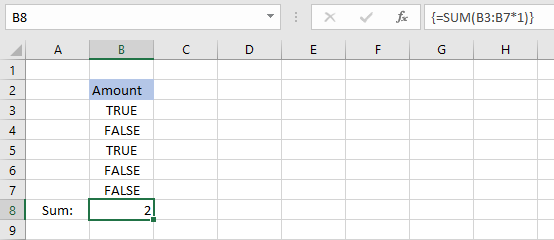The formula returns 2 because TRUE equals 1 and FALSE equals 0. 1+0+1+0+0 = 2.

Note, you can use the SUMPRODUCT function if you don't want to use array formulas.

Regular formula in cell B8:

=SUMPRODUCT(B3:B7*1)

## 7. How to create a running total?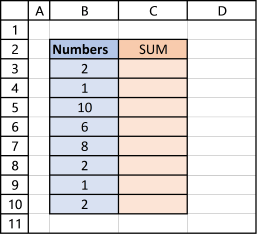The image above shows you numbers in column B.

Enter this formula in cell C3:

=SUM(\$B\$3:B3)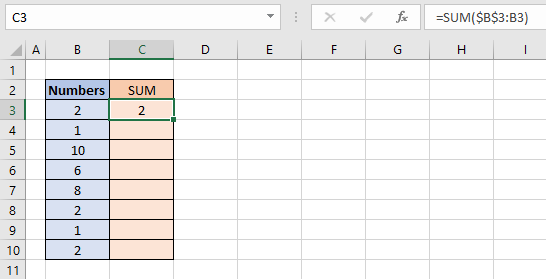Make sure you get the dollar signs right, they are important. The cell reference changes as you copy the formula and paste it to cells below.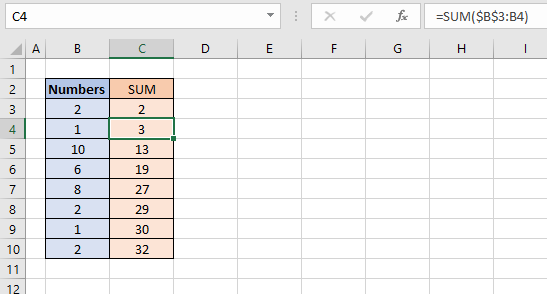Select cell C4 and see how the formula changed in the formula bar. The part of the cell reference without dollar signs changed from B3 to B4.

That part is a relative cell reference and the part with dollar signs is an absolute cell reference.
Read more here: Absolute and relative cell references

This makes the SUM function use a cell reference that grows, in other words, it includes more and more cells creating a running total.

Formula in cell C4 adds numbers from both cell B3 and B4. The formula grows even further in cell C5 and it keeps growing in cells below.

Note, you can double press with left mouse button on the dot in the lower right corner of the cell to automatically copy the cell and paste it to cells below as far as there are populated cells in the adjacent column.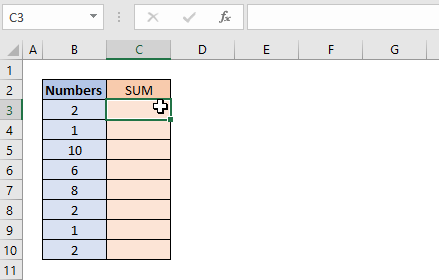## 8. How to sum with a condition [array formula]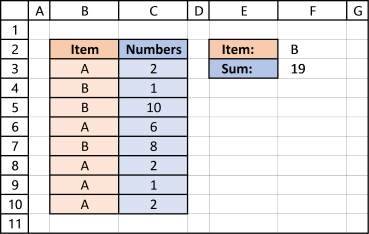The picture above shows you two columns, column B contains text values and column C contains numbers. The formula demonstrated here allows you to sum by another column.

The formula in cell F3 lets you add numbers in column C if their adjacent value is equal to the value in cell F2:

=SUM((B3:B10=F2)*C3:C10)

This formula is entered as an array formula unless you are using Excel 365. I recommend the SUMIF function built exactly for this without entering the formula as an array formula.

### Explaining formula in cell F3

#### Step 1 - Logical expression

The equal sign in B3:B10=F2 lets you compare the values in cell range B3:B10 with the value in cell F2. The equal sign is a logical operator, often used in IF functions.

{"A";"B";"B";"A";"B";"A";"A";"A"}="B"

This logical test returns an array of boolean values:

{FALSE; TRUE; TRUE; FALSE; TRUE; FALSE; FALSE; FALSE}

#### Step 2 - Multiply array with cell range

The parentheses (B3:B10=F2) make sure this part of the formula is calculated first before multiplying with the numbers in cell range C3:C10.

(B3:B10=F2)*C3:C10

becomes

{FALSE; TRUE; TRUE; FALSE; TRUE; FALSE; FALSE; FALSE}*C3:C10

becomes

{FALSE; TRUE; TRUE; FALSE; TRUE; FALSE; FALSE; FALSE}*{2; 1; 10; 6; 8; 2; 1; 2}

FALSE is equal to 0 (zero) and TRUE is equal to 1.

{FALSE; TRUE; TRUE; FALSE; TRUE; FALSE; FALSE; FALSE}*{2; 1; 10; 6; 8; 2; 1; 2}

becomes

{0; 1; 1; 0; 1; 0; 0; 0}*{2; 1; 10; 6; 8; 2; 1; 2}

becomes

{0*2;1*1;1*10;0*6;1*8;0*2;0*1;0*2}

and returns

{0; 1; 10; 0; 8; 0; 0; 0}

#### Step 3 - Sum numbers

The SUM function then adds the number in the array:

SUM({0; 1; 10; 0; 8; 0; 0; 0})

and returns 19 in cell F3. 1+10+8 = 19

Tip! The powerful Excel table can do all this for you:
Excel defined Table - SUM with criteria

## 9. Sum with multiple conditions [array formula]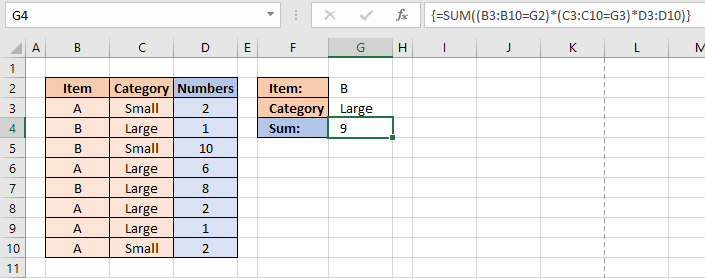Adding a second condition to the formula is easy. Simply add your condition to the formula enclosed with parentheses.

=SUM((B3:B10=G2)*(C3:C10=G3)*D3:D10)

This formula is entered as an array formula unless you are using Excel 365. I recommend the SUMIFS function built exactly for this without the need for an array formula.

### Explaining formula in cell G4

#### Step 1 - First condition

The equal sign allows you to compare cells to each other.

B3:B10=G2

becomes

{"A";"B";"B";"A";"B";"A";"A";"A"}="B"

and returns

{FALSE; TRUE; TRUE; FALSE; TRUE; FALSE; FALSE; FALSE}

#### Step 2 - Second condition

C3:C10=G3

becomes

{"Small";"Large";"Small";"Large";"Large";"Large";"Large";"Small"}="Large"

and returns

{FALSE; TRUE; FALSE; TRUE; TRUE; TRUE; TRUE; FALSE}

#### Step 3 - Multiply arrays

(B3:B10=G2)*(C3:C10=G3)*D3:D10

becomes

{FALSE; TRUE; TRUE; FALSE; TRUE; FALSE; FALSE; FALSE}*{FALSE; TRUE; FALSE; TRUE; TRUE; TRUE; TRUE; FALSE}*{2;1;10;6;8;2;1;2}

becomes

{0; 1; 0; 0; 1; 0; 0; 0}*{2; 1; 10; 6; 8; 2; 1; 2}

and returns

{0;1;0;0;8;0;0;0}

#### Step 4 - Sum values in the array

SUM((B3:B10=G2)*(C3:C10=G3)*D3:D10)

becomes

SUM({0;1;0;0;8;0;0;0})

and returns 9.

The SUMPRODUCT function allows you to perform the same calculation without the need for entering the formula as an array formula.

=SUMPRODUCT((B3:B10=G2)*(C3:C10=G3)*D3:D10)

## 10. How to sum only visible cells?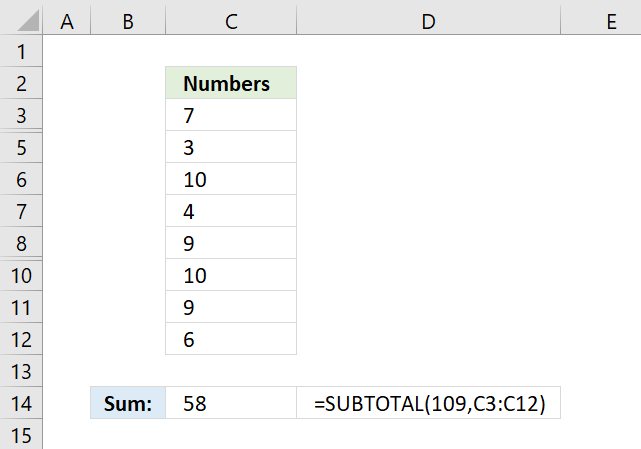The SUBTOTAL function lets you sum values in a cell range that have some rows hidden or filtered, the picture above shows a cell range that has row 4 and 9 hidden. The usual SUM function won't work in this case, you need the SUBTOTAL function:

=SUBTOTAL(109,C3:C12)

The first argument allows you to pick a function number that determines how the SUBTOTAL function behaves. In this case, 109 sums all visible cells in a cell range.

To hide a value simply press with right mouse button on on a row number and then press with left mouse button on "Hide" to hide the entire row. Select the rows around a hidden row and then press with right mouse button on on them to open a menu, there press with left mouse button on "Unhide" to show the value again.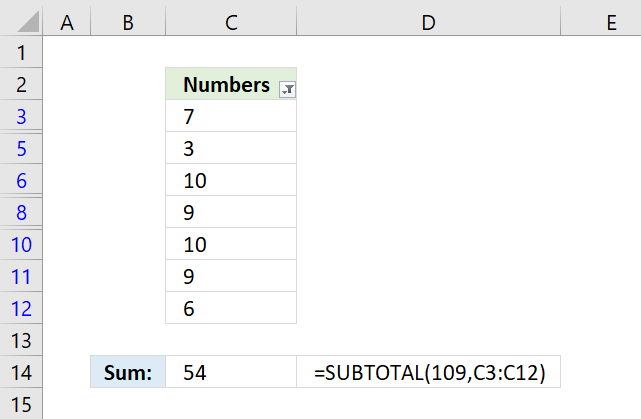The picture above shows filtered values in column C. Excel tells you that the cell range is filtered by the color of the row numbers and the icon next to "Numbers" in cell C2.

To apply a filter to a column simply select the cell range, go to tab "Data" on the ribbon, press with left mouse button on "Filter" button. A black down-pointing arrow appears next to header name "Numbers", press with left mouse button on it to apply a filter.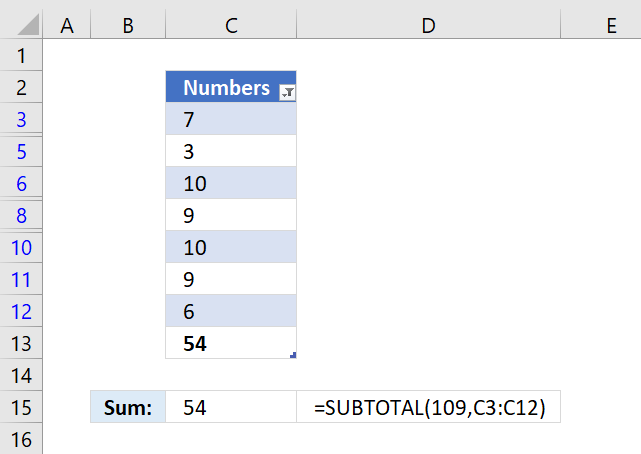The Excel defined table above has a built-in feature that allows you to sum filtered values automatically, all you need to do is select a cell in the table, go to tab "Design" on the ribbon, then press with left mouse button on check-box "Total Row" to show the totals.

Cell C13 in the picture above displays the total for filtered cells. The SUBTOTAL function works just as well if you prefer using an Excel function with an Excel defined table, demonstrated in cell C15.

How to hide / unhide values?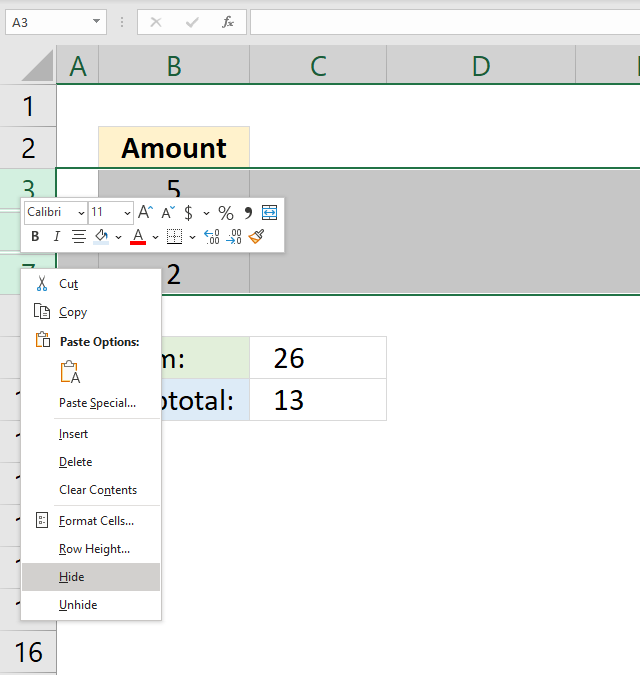Note, follow these instructions on how to hide and unhide specific rows:

1. Press with right mouse button on on row number.
2. A popup-menu appears, see image above.
3. Press with mouse on Hide or Unhide.

Tip! Press and hold CTRL key while selecting rows to hide/unhide multiple values at the same time.

## 11. How to sum a filtered column?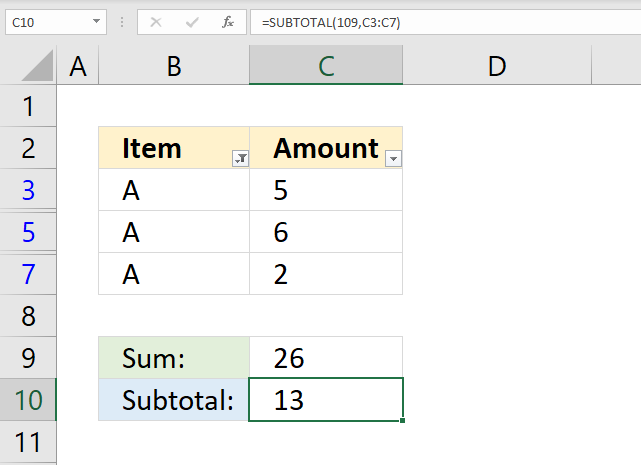The image above shows numbers in cell range C3:C7, however, a filter is applied and rows 4 and 6 are hidden. The SUM function in cell C9 can't ignore filtered values, you need the SUBTOTAL function to sum filtered numbers.

Formula in cell C10:

=SUBTOTAL(109, B3:B7)

## 12. How to sum the entire column?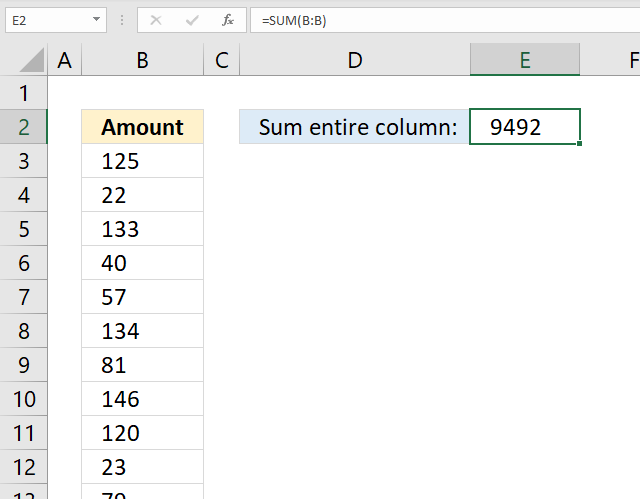The image above shows a formula that adds all values in a column and returns a total.

Formula in cell E2:

=SUM(B:B)

The cell reference is B:B meaning that all numerical values in column B are included in the total.

## 13. How to sum a row?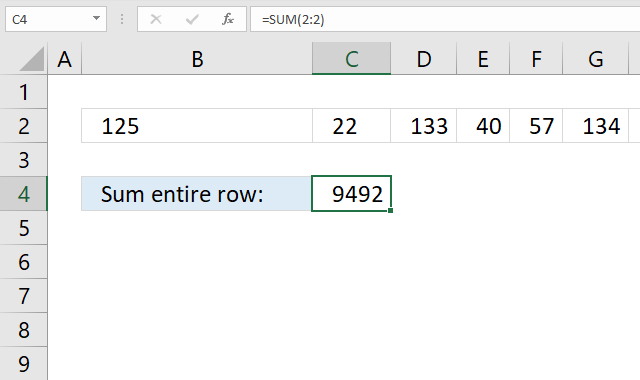The image above shows a formula that adds all values in a row and returns a total.

Formula in cell C4:

=SUM(2:2)

The cell reference is 2:2 meaning that all numerical values in row 2 are included in the total.

## 14. How to sum across worksheets?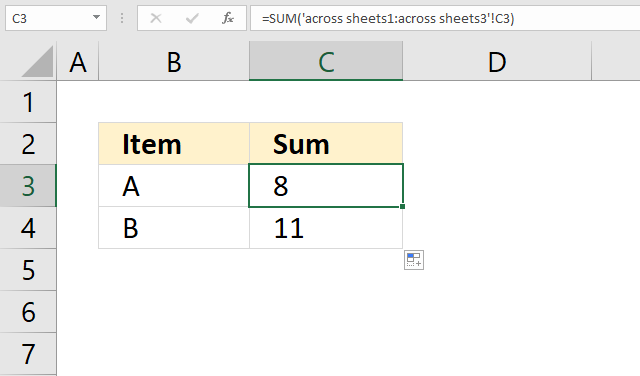The image above shows a formula that adds values located in cell C3 across three different worksheets. For this to work values you want to add must be located in the same cell across all worksheets.

Formula in cell C3:

=SUM('across sheets1:across sheets3'!C3)

Here are the steps I did to create the formula above:

1. Doublepress with left mouse button on cell C3, the prompt is shown.
2. Type =SUM(
3. Go to the first worksheet.
4. Press with mouse on the cell containing the value you want to add.
5. Press and hold SHIFT key.
6. Select the last worksheet you want to include in the total.
7. Release the SHIFT key.
8. Type an ending parenthesis )
9. Press Enter.

The image below shows the tabs I selected to create the formula above.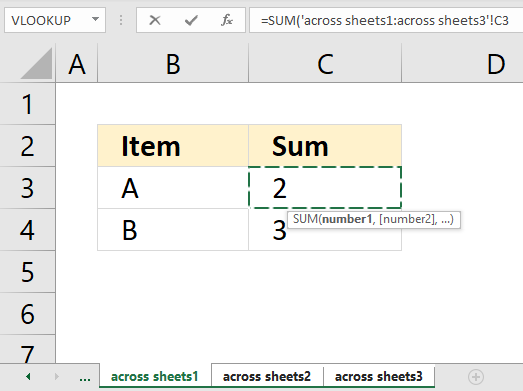## 15. How to sum by color?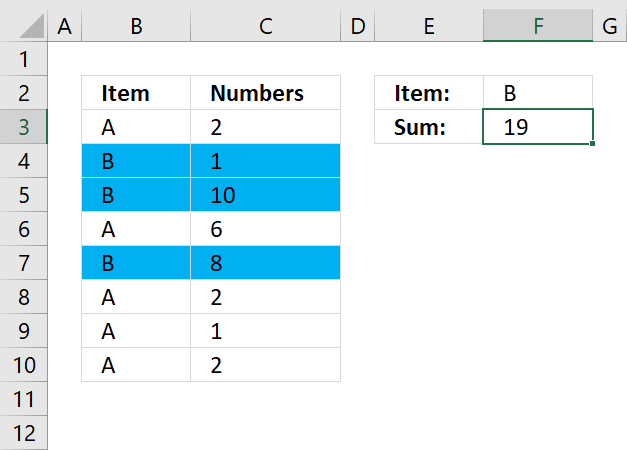The short answer is that there is really no way to sum by background color if you want to use formulas, however, a VBA macro can do it.

The long answer is that there is the GET.CELL function that has some serious flaws, one is that it is outdated and may be removed from Excel by Microsoft whenever they feel like it. I'd rather recommend coloring cells using Conditional Formatting and then using the same condition to sum the cells.

This is what the image above shows, I chose to highlight rows blue if the corresponding cell in column B is equal to item "B". Here is how I did it:

1. Select the cells you want to highlight, in the example above cell range B3:C10.
2. Go to tab "Home" on the ribbon.
3. Press with left mouse button on the Conditional Formatting button.
4. Press with left mouse button on "New Rule...". A dialog box appears.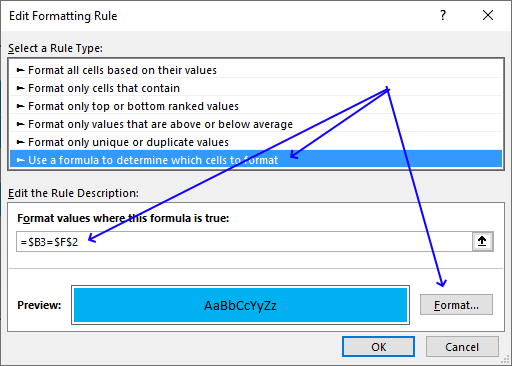5. Press with mouse on "Use a formula to determine which cells to format"
6. Press with mouse on field below "Format values where this formula is true:".
7. Type =\$B3=\$F\$2
8. Press with left mouse button on "Format..." button. A new dialog box appears.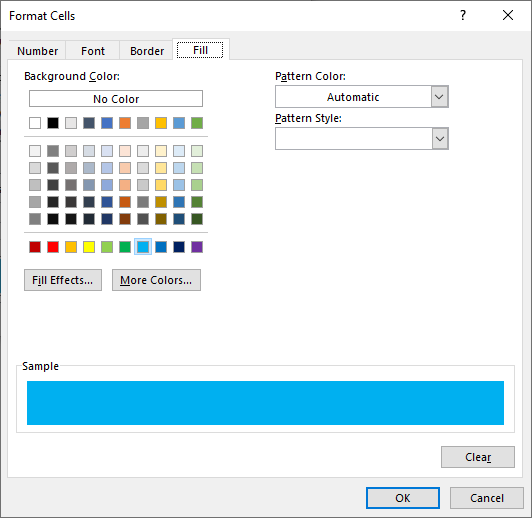9. Press with left mouse button on tab "Fill" on top menu.
10. Pick a color.
11. Press with left mouse button on OK. The dialog box is closed.
12. Press with left mouse button on OK again.The formula in cell F3 is explained here: How to sum numbers based on a condition/label/item/category?

## 16. How to sum absolute numbers?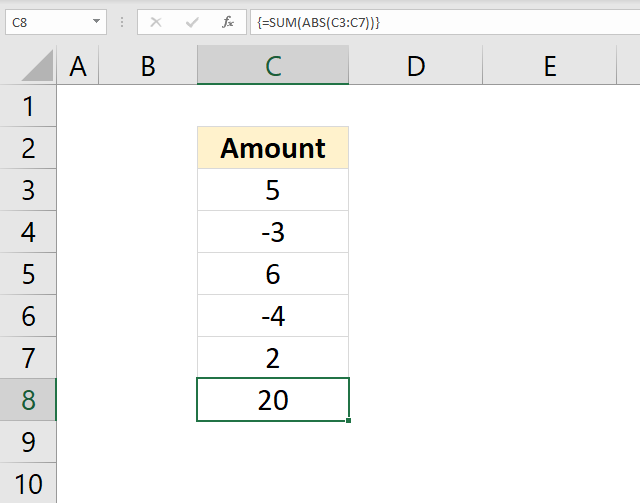The image above shows a formula in cell C8 that converts negative values to positive values and then adds the values.

Formula in cell C8:

=SUM(ABS(C3:C7))

### Explaining formula in cell C8

#### Step 1 - Remove sign

The ABS function converts negative numbers to positive numbers, in other words, the ABS function removes the sign.

ABS(number)

ABS(C3:C7)

becomes

ABS({5; -3; 6; -4; 2})

and returns

{5; 3; 6; 4; 2}

#### Step 2 - Add values

SUM(ABS(C3:C7))

becomes

SUM({5; 3; 6; 4; 2})

and returns 20. 5+3+6+4+2 = 20

## 17. How to use the SUM function in a macro (VBA)?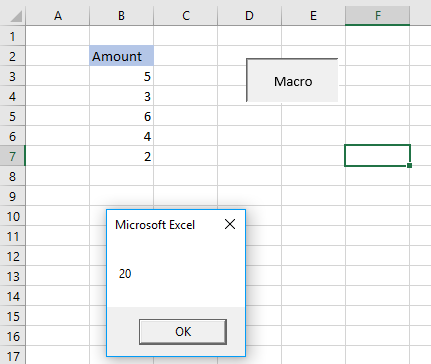The image above demonstrates a macro that shows a message box with a number representing the total of cell range B3:B7.

```'Macro name
Sub HLP()

'Show sum of B3:B7 in a messagebox
MsgBox Application.WorksheetFunction.Sum(Range("B3:B7"))

'Exit macro
End Sub
```

Microsoft docs: | Application.WorksheetFunction | Sum | Range | Msgbox

## 18. What is the shortcut key for the SUM function?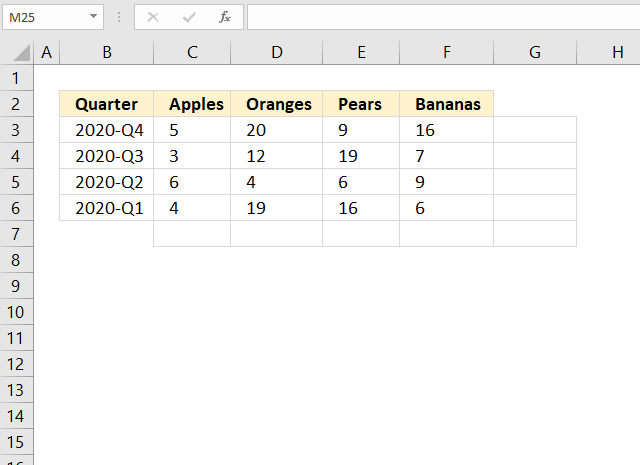The animated image above shows how to add totals for a cell range, both vertically and horizontally, using a shortcut key.

The SUM formulas in cell range G3:G7 adds values from the cell to the left of the formula and on the same row.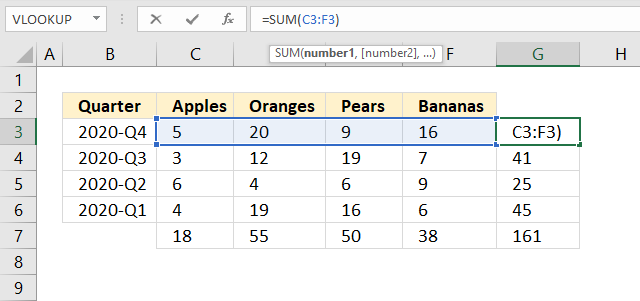The SUM formulas in cell range C7:F7 return a total based on the numbers above the formulas in the same column.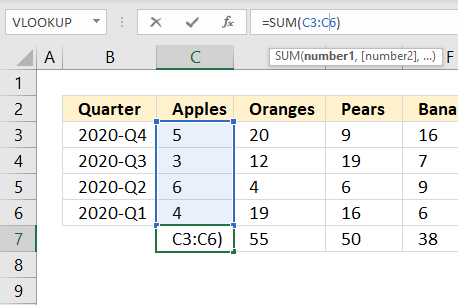Here is how to create the SUM function using a shortcut key:

1. Select the cell range containing numbers.
2. Press and hold Alt key.
3. Press the equal sign =
4. Release the Alt key.

If the steps above don't work for you try Alt + Shift + 0 (zero) keys. It really depends on your keyboard layout which keys you need to press.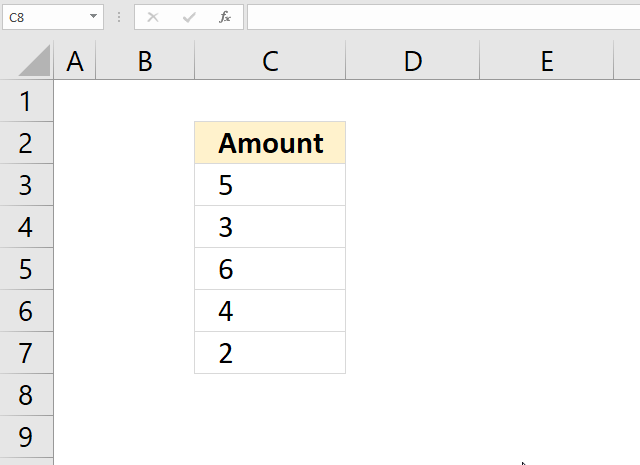The ímage above shows that you can use the shortcut key below numbers in a column.

## 19. How to sum values below/above a threshold?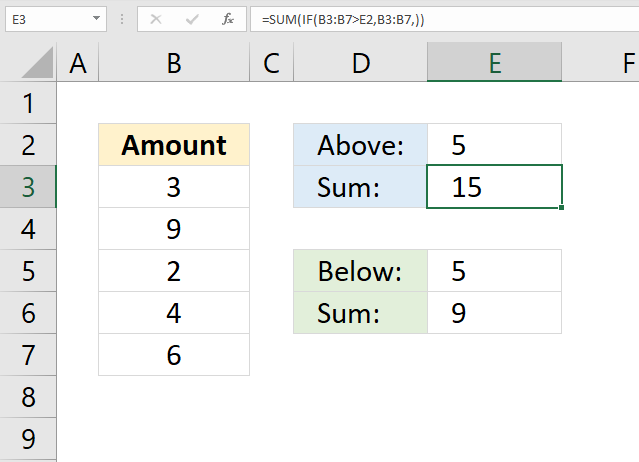The image above demonstrates two array formulas in cell E3 and E5 that return a total with values larger or smaller than a given threshold.

Array formula in cell E3:

=SUM(IF(B3:B7>E2, B3:B7, ))

Array formula in cell E6:

=SUM(IF(B3:B7<E2,B3:B7,))

How to enter an array formula

I recommend the SUMIF function built exactly for this without entering the formula as an array formula.

### Explaining formula in cell E3

#### Step 1 - Filter values above a threshold

The IF function returns one value if the logical test is TRUE and another value if the logical test is FALSE.

IF(logical_test, [value_if_true], [value_if_false])

IF(B3:B7>E2, B3:B7, )

becomes

IF({3;9;2;4;6}>5,{3;9;2;4;6},)

becomes

IF({FALSE; TRUE; FALSE; FALSE; TRUE},{3; 9; 2; 4; 6},)

and returns

{0;9;0;0;6}

#### Step 2 - Sum values

SUM(IF(B3:B7>E2, B3:B7, ))

becomes

SUM({0;9;0;0;6})

and returns 15 in cell E3.

##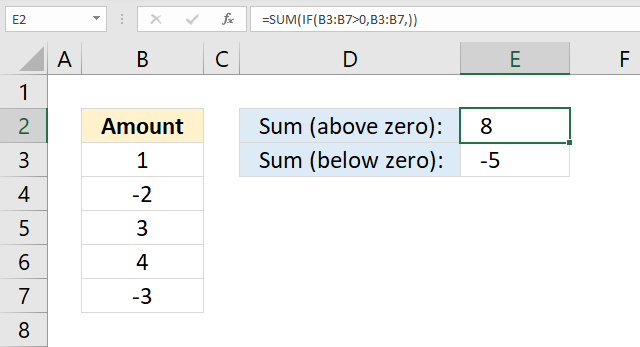The image above demonstrates two formulas in cells E2 and E3 that return a total with values above and below zero respectively.

Array formula in cell E2:

=SUM(IF(B3:B7>0, B3:B7, ))

Array formula in cell E3:

=SUM(IF(B3:B7<0, B3:B7, ))

How to enter an array formula

I recommend the SUMIF function built exactly for this without entering the formula as an array formula.

### Explaining formula in cell E2

#### Step 1 - Filter values above a 0 (zero)

The IF function returns one value if the logical test is TRUE and another value if the logical test is FALSE.

IF(logical_test, [value_if_true], [value_if_false])

IF(B3:B7>0, B3:B7, )

becomes

IF({1;-2;3;4;-3}>0,{1;-2;3;4;-3},)

becomes

IF({TRUE;FALSE;TRUE;TRUE;FALSE},{1;-2;3;4;-3},)

and returns

{1;0;3;4;0}

#### Step 2 - Sum numbers

SUM(IF(B3:B7>0, B3:B7, ))

becomes

SUM({1;0;3;4;0})

and returns 8 in cell E2. 1 + 3 + 4 = 8.

## 21. How to limit the SUM function?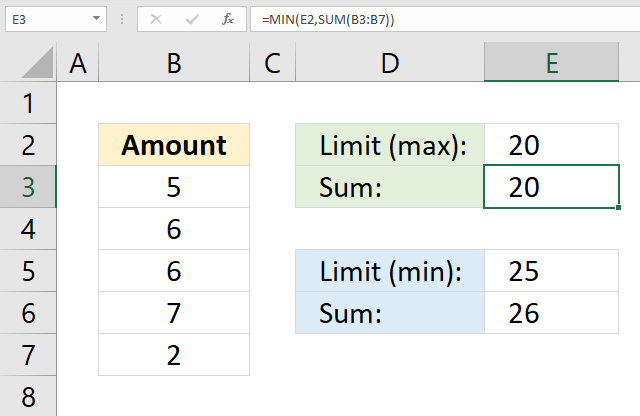The image above demonstrates a formula in cell E3 that sums values in cell range B3:B7 and returns a total that is limited to the number specified in cell E2. In other words, the total can't be larger than the value in cell E2 but it can be smaller.

The image above also shows a formula in cell E6 that adds values in cell range B3:B7 and returns a total that is limited to the number specified in cell E5. The total can't be smaller than the value in cell E5 but it can be larger.

Formula in cell E3:

=MIN(E2, SUM(B3:B7))

Formula in cell E6:

=MAX(E5, SUM(B3:B7))

### Explaining formula in cell E3

#### Step 1 - Sum numbers

SUM(B3:B7)

becomes

SUM({5;6;6;7;2})

and returns 26. 5+6+6+7+2=26

#### Step 2 - Return the smallest number

The MIN function returns the smallest number in a cell range or array.

MIN(E2, SUM(B3:B7))

becomes

MIN(20, 26)

and returns 20. 20 is smaller than 26.

## 22. How to sum a column and ignore errors?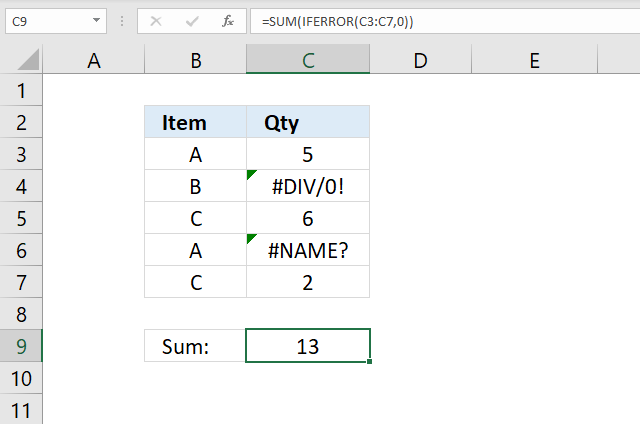The image above shows a formula in cell C9 that sums numbers in cell range C3:C7 and ignores errors.

Array Formula in cell C9:

=SUM(IFERROR(C3:C7, 0))

### Explaining formula in cell C9

#### Step 1 - Replace errors with 0 (zero)

The IFERROR function lets you handle most formula errors with ease.

IFERROR(valuevalue_if_error)

IFERROR(C3:C7, 0)

becomes

IFERROR({5;#DIV/0!;6;#NAME?;2})

and returns

{5;0;6;0;2}

#### Step 2 - Sum values in array

SUM(IFERROR(C3:C7, 0))

becomes

SUM({5;0;6;0;2})

and returns 13 in cell C9. 5+6+2=13

Note that the IFERROR catches all errors and may cause problems if you want to troubleshoot formulas. Why? You can't find errors.

## 23. Sum values based on a date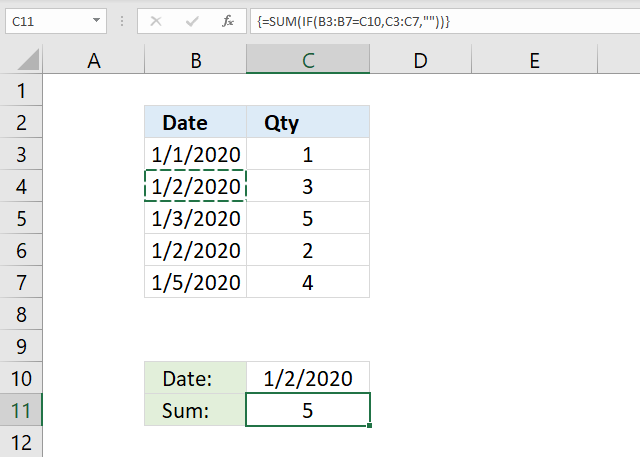The image above shows a formula in cell C11 that sums numbers in cell range C3:C7 if dates in B3:B7 are equal to C10.

Array formula in cell C11:

=SUM(IF(B3:B7=C10, C3:C7, ""))

I recommend the SUMIF function built exactly for this without entering the formula as an array formula.

### Explaining formula in cell C9

#### Step 1 - Logical expression

B3:B7=C10

becomes

{43831;43832;43833;43832;43835}=43832

and returns

{FALSE; TRUE; FALSE; TRUE; FALSE}

#### Step 2 - Evaluate IF function

The IF function returns one value if the logical test is TRUE and another value if the logical test is FALSE.

IF(B3:B7=C10, C3:C7, "")

becomes

IF({FALSE; TRUE; FALSE; TRUE; FALSE}, C3:C7, "")

becomes

IF({FALSE; TRUE; FALSE; TRUE; FALSE}, {1; 3; 5; 2; 4},  "")

and returns {""; 3; ""; 2; ""}.

#### Step 3 - Sum values in the array

SUM(IF(B3:B7=C10, C3:C7, ""))

becomes

SUM({""; 3; ""; 2; ""})

and returns 5. 3+2 = 5.

## 24. Sum values based on week number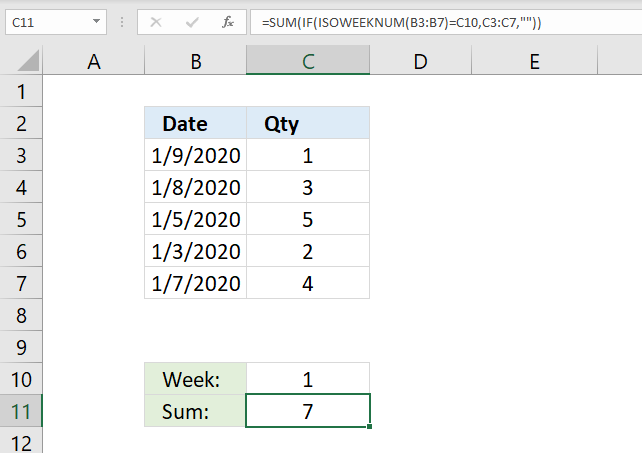The image above shows a formula in cell C11 that sums numbers in cell range C3:C7 if the corresponding weeks based on the dates in B3:B7 are equal to C10.

Array formula in cell C11:

=SUM(IF(ISOWEEKNUM(B3:B7)=C10, C3:C7, ""))

I recommend the SUMIF function built exactly for this without entering the formula as an array formula.

### Explaining formula in cell C9

#### Step 1 - Calculate week numbers

The ISOWEEKNUM function calculates the number of the ISO week number of the year for a specific date.

ISOWEEKNUM(B3:B7)

becomes

ISOWEEKNUM({43839; 43838; 43835; 43833; 43837})

and returns {2; 2; 1; 1; 2}

#### Step 2 - Logical expression

ISOWEEKNUM(B3:B7)=C10

becomes

{2; 2; 1; 1; 2}=1

and returns {FALSE; FALSE; TRUE; TRUE; FALSE}.

#### Step 3 - Evaluate IF function

The IF function returns one value if the logical test is TRUE and another value if the logical test is FALSE.

IF(ISOWEEKNUM(B3:B7)=C10, C3:C7, "")

becomes

IF({FALSE; FALSE; TRUE; TRUE; FALSE}, C3:C7, "")

becomes

IF({FALSE; FALSE; TRUE; TRUE; FALSE}, {1; 3; 5; 2; 4}, "")

and returns {""; ""; 5; 2; ""}

#### Step 4 - Sum values in the array

SUM(IF(ISOWEEKNUM(B3:B7)=C10, C3:C7, ""))

becomes

SUM({""; ""; 5; 2; ""})

and returns 7. 5+2 = 7.

## 25. Sum values by month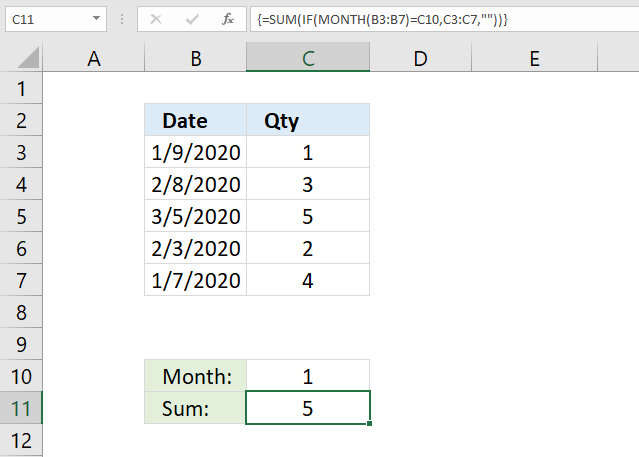The image above shows a formula in cell C11 that sums numbers in cell range C3:C7 if the corresponding weeks based on the dates in B3:B7 are equal to C10.

Array formula in cell C11:

=SUM(IF(MONTH(B3:B7)=C10, C3:C7, ""))

I recommend the SUMIF function built exactly for this without entering the formula as an array formula.

### Explaining formula in cell C9

#### Step 1 - Calculate number representing the position of the month in a year

The MONTH function extracts the month as a number from an Excel date.

1 - January, 2 - February, 3 - March, 4 - April, 5 - May, 6 - June, 7 - July, 8 - August, 9 - September, 10 - October, 11 - November, 12 - December

MONTH(B3:B7)

becomes

MONTH({43839; 43869; 43895; 43864; 43837})

returns

{1; 2; 3; 2; 1}

#### Step 2 - Logical expression

MONTH(B3:B7)=C10

becomes

{1; 2; 3; 2; 1}=1

and returns {TRUE; FALSE; FALSE; FALSE; TRUE}

#### Step 3 - Evaluate IF function

The IF function returns one value if the logical test is TRUE and another value if the logical test is FALSE.

IF(MONTH(B3:B7)=C10, C3:C7, "")

becomes

IF({TRUE; FALSE; FALSE; FALSE; TRUE}, {1; 3; 5; 2; 4}, "")

and returns {1; ""; ""; ""; 4}

#### Step 4 - Sum values in the array

SUM(IF(MONTH(B3:B7)=C10, C3:C7, ""))

becomes

SUM({1; ""; ""; ""; 4})

and returns 5. 1 + 4 = 5.

## 26. Sum values by year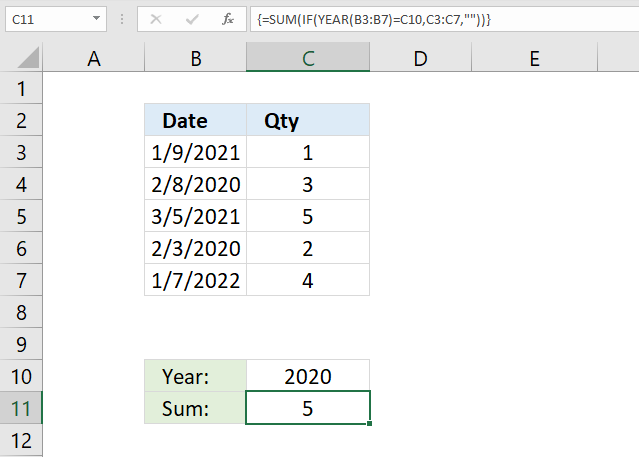The image above shows a formula in cell C11 that sums numbers in cell range C3:C7 if the corresponding weeks based on the dates in B3:B7 are equal to C10.

Array formula in cell C11:

=SUM(IF(YEAR(B3:B7)=C10, C3:C7, ""))

I recommend the SUMIF function built exactly for this without entering the formula as an array formula.

### Explaining formula in cell C9

#### Step 1 - Calculate year based on an Excel date

The YEAR function returns the year from an Excel date.

YEAR(B3:B7)

becomes

YEAR({44205; 43869; 44260; 43864; 44568})

and returns {2021; 2020; 2021; 2020; 2022}

#### Step 2 - Logical expression

YEAR(B3:B7)=C10

becomes

{2021; 2020; 2021; 2020; 2022}=2020

and returns {FALSE; TRUE; FALSE; TRUE; FALSE}

#### Step 3 - Evaluate IF function

The IF function returns one value if the logical test is TRUE and another value if the logical test is FALSE.

IF(YEAR(B3:B7)=C10, C3:C7, "")

becomes

IF({FALSE; TRUE; FALSE; TRUE; FALSE}, {1; 3; 5; 2; 4}, "")

and returns {""; 3; ""; 2; ""}

#### Step 4 - Sum values in array

SUM(IF(YEAR(B3:B7)=C10, C3:C7, ""))

becomes

SUM({""; 3; ""; 2; ""})

and returns 5. 3 + 2 = 5.

## 27. Sum values between two dates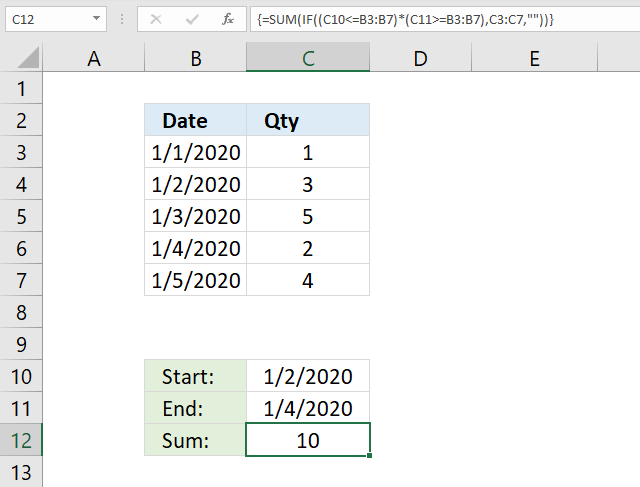The image above shows a formula in cell C12 that sums numbers in cell range C3:C7 if dates in B3:B7 are less than or equal to C11 and greater than or equal to C10.

Array formula in cell C12:

=SUM(IF((C10<=B3:B7)*(C11>=B3:B7), C3:C7, ""))

I recommend the SUMIFS function built exactly for this without entering the formula as an array formula.

### Explaining formula in cell C9

#### Step 1 - First logical expression

C10<=B3:B7

becomes

43832<={43831; 43832; 43833; 43834; 43835}

and returns

{FALSE; TRUE; TRUE; TRUE; TRUE}

#### Step 2 - Second logical expression

C11>=B3:B7

becomes

43834>={43831;43832;43833;43834;43835}

and returns

{TRUE; TRUE; TRUE; TRUE; FALSE}

#### Step 3 - Multiply arrays

(C10<=B3:B7)*(C11>=B3:B7)

becomes

{FALSE; TRUE; TRUE; TRUE; TRUE}*{TRUE; TRUE; TRUE; TRUE; FALSE}

and returns {0; 1; 1; 1; 0}

#### Step 4 - Evaluate IF function

The IF function returns one value if the logical test is TRUE and another value if the logical test is FALSE.

IF((C10<=B3:B7)*(C11>=B3:B7), C3:C7, "")

becomes

IF({0; 1; 1; 1; 0}, C3:C7, "")

becomes

IF({0; 1; 1; 1; 0}, {1; 3; 5; 2; 4}, "")

and returns {""; 3; 5; 2; ""}

#### Step 5 - Sum values in the array

SUM(IF((C10<=B3:B7)*(C11>=B3:B7), C3:C7, ""))

becomes

SUM({""; 3; 5; 2; ""})

and returns 10. 3+5+2 = 10.